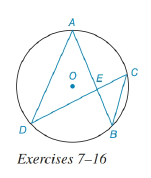Chapter 6.3, Problem 7EElementary Geometry For College St...

7th Edition
Alexander + 2 others
ISBN: 9781337614085

Solutions

Chapter
SectionElementary Geometry For College St...

7th Edition
Alexander + 2 others
ISBN: 9781337614085
Textbook Problem

In the figure for Exercises 7 to 16, O is the center of the circle. See Theorem 6.3.5.Given: A E = 6 , E B = 4 , D E = 8 Find: EC.To determine

To find:

To find EC.

Explanation

Given that AE=6,EB=4,DE=8.

The diagrammatic representation is given below,

Theorem:

If two chords intersect within a circle, then the product of the lengths of the segments (parts) of one chord is equal to the product of the lengths of the segments of the other chord.

By using the theorem to get the following,

AEEB=DEEC

Substitute the values AE=6,EB=4,DE=8 in the above equation to get the following,

Still sussing out bartleby?

Check out a sample textbook solution.

See a sample solution

The Solution to Your Study Problems

Bartleby provides explanations to thousands of textbook problems written by our experts, many with advanced degrees!

Get Started

Evaluate limx1x31x1.

Single Variable Calculus

Find the x- and y-intercepts of the graph of y=x22x3.

Calculus: An Applied Approach (MindTap Course List)

If P=2a+b,a=36,andb=15,findP.

Elementary Technical Mathematics

converges to: 2 the series diverges

Study Guide for Stewart's Multivariable Calculus, 8th

Find for x = 3t2 + 1, y = t6 + 6t5. t4 + 5t3 4t3 + 15t2

Study Guide for Stewart's Single Variable Calculus: Early Transcendentals, 8th